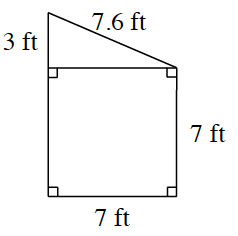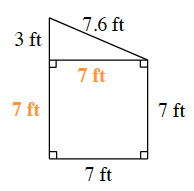### Home > ACC6 > Chapter 4 Unit 11 > Lesson CC2: 4.2.4 > Problem4-62

4-62.

Find the area and perimeter of the shape at right.To find the area, treat this shape as a square and a right triangle.
Find their individual areas, and then add them together.

$(7·7)+(3·7\div2)=?$

To find the perimeter, add together all of the side lengths.

$3+7.6+7+7+7+?$

Area: $59.5$ square feet
Perimeter: $31.6$ feet